1607501400

# How to Build a Website- Full Step by Step Tutorial

Learn how to build a website for beginners. This is a full tutorial on building a website step by step. This video is a tutorial on building a website from scratch with Wix. Mark Lassoff will take you step-by-step and how you everything you need to know on how to build a web site. This video is designed for beginners, so no prior knowledge is needed to build your very first website with Wix.

Contents
00:00 Opening
02:34 Touring Wix Features
06:35 The Wix Editor Toolbar
07:53 Managing Multiple Pages
10:29 Creating the Project Site
11:13 Page Backgrounds
13:06 The Parts of a Webpage (Header, Footer)
15:48 Working with Images
17:31 Working with Site Text
20:46 Wix Videomaker Feature
24:30 Lists and Grids
27:58 Search Engine Optimization Features
31:33 Goodbye from Mark

#web-development #html #css #javascript

## Buddha Community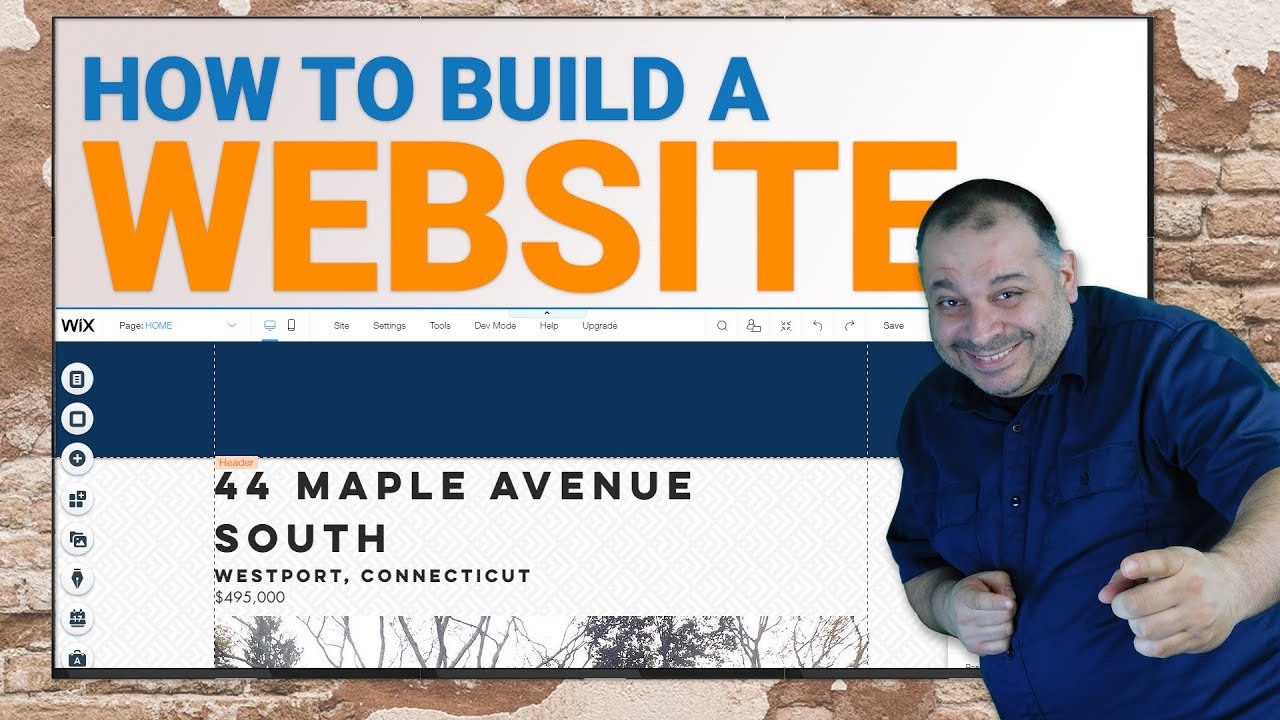1561523460

## Matplotlib Cheat Sheet: Plotting in Python

This Matplotlib cheat sheet introduces you to the basics that you need to plot your data with Python and includes code samples.

Data visualization and storytelling with your data are essential skills that every data scientist needs to communicate insights gained from analyses effectively to any audience out there.

For most beginners, the first package that they use to get in touch with data visualization and storytelling is, naturally, Matplotlib: it is a Python 2D plotting library that enables users to make publication-quality figures. But, what might be even more convincing is the fact that other packages, such as Pandas, intend to build more plotting integration with Matplotlib as time goes on.

However, what might slow down beginners is the fact that this package is pretty extensive. There is so much that you can do with it and it might be hard to still keep a structure when you're learning how to work with Matplotlib.

DataCamp has created a Matplotlib cheat sheet for those who might already know how to use the package to their advantage to make beautiful plots in Python, but that still want to keep a one-page reference handy. Of course, for those who don't know how to work with Matplotlib, this might be the extra push be convinced and to finally get started with data visualization in Python.

You'll see that this cheat sheet presents you with the six basic steps that you can go through to make beautiful plots.

Check out the infographic by clicking on the button below: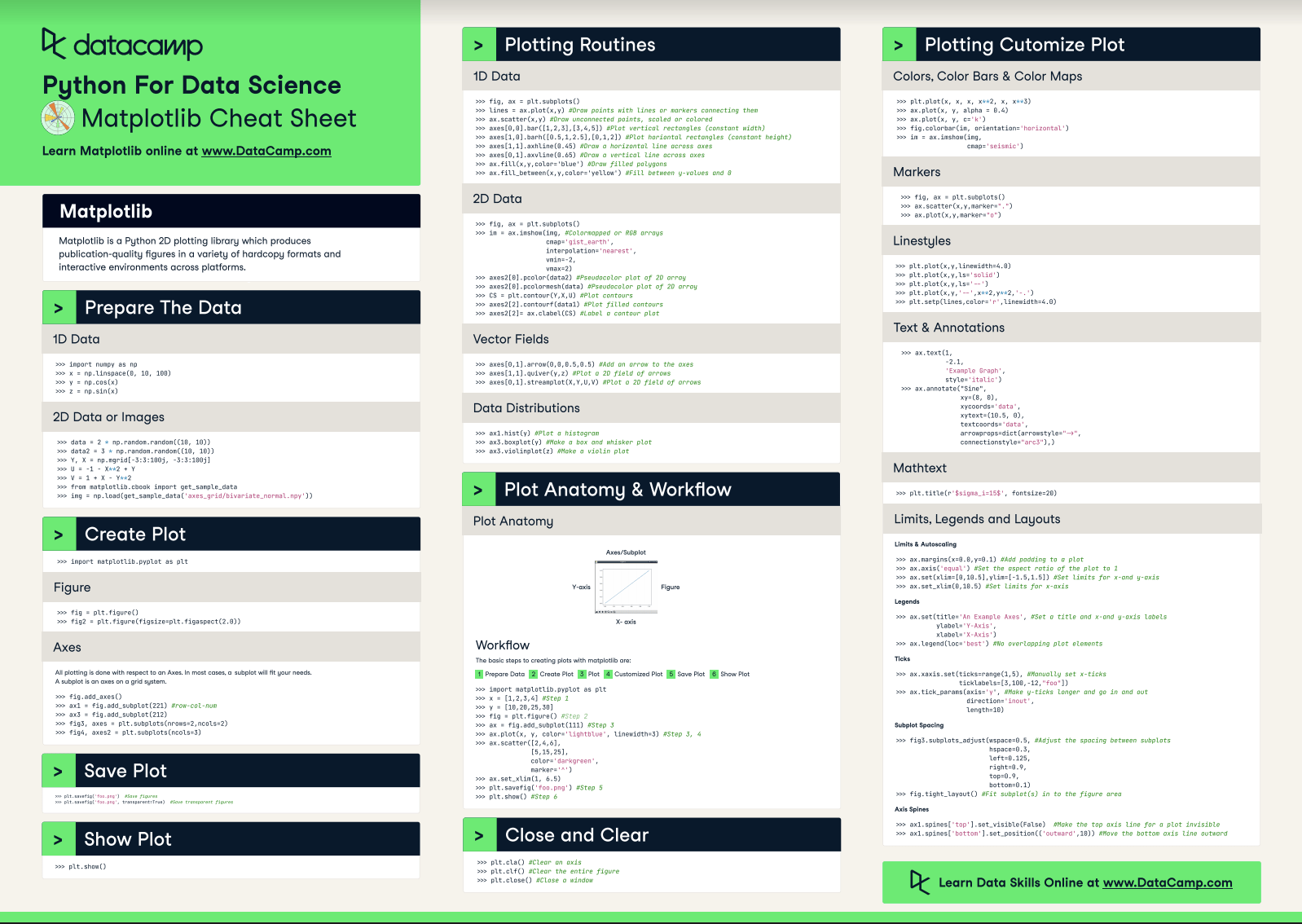With this handy reference, you'll familiarize yourself in no time with the basics of Matplotlib: you'll learn how you can prepare your data, create a new plot, use some basic plotting routines to your advantage, add customizations to your plots, and save, show and close the plots that you make.

What might have looked difficult before will definitely be more clear once you start using this cheat sheet! Use it in combination with the Matplotlib Gallery, the documentation.

Matplotlib

Matplotlib is a Python 2D plotting library which produces publication-quality figures in a variety of hardcopy formats and interactive environments across platforms.

## Prepare the Data

### 1D Data

``````>>> import numpy as np
>>> x = np.linspace(0, 10, 100)
>>> y = np.cos(x)
>>> z = np.sin(x)``````

### 2D Data or Images

``````>>> data = 2 * np.random.random((10, 10))
>>> data2 = 3 * np.random.random((10, 10))
>>> Y, X = np.mgrid[-3:3:100j, -3:3:100j]
>>> U = 1 X** 2 + Y
>>> V = 1 + X Y**2
>>> from matplotlib.cbook import get_sample_data

## Create Plot

``>>> import matplotlib.pyplot as plt``

### Figure

``````>>> fig = plt.figure()
>>> fig2 = plt.figure(figsize=plt.figaspect(2.0))``````

### Axes

``````>>> fig.add_axes()
>>> fig3, axes = plt.subplots(nrows=2,ncols=2)
>>> fig4, axes2 = plt.subplots(ncols=3)``````

## Save Plot

``````>>> plt.savefig('foo.png') #Save figures
>>> plt.savefig('foo.png',  transparent=True) #Save transparent figures``````

## Show Plot

``>>> plt.show()``

## 1D Data

``````>>> fig, ax = plt.subplots()
>>> lines = ax.plot(x,y) #Draw points with lines or markers connecting them
>>> ax.scatter(x,y) #Draw unconnected points, scaled or colored
>>> axes[0,0].bar([1,2,3],[3,4,5]) #Plot vertical rectangles (constant width)
>>> axes[1,0].barh([0.5,1,2.5],[0,1,2]) #Plot horiontal rectangles (constant height)
>>> axes[1,1].axhline(0.45) #Draw a horizontal line across axes
>>> axes[0,1].axvline(0.65) #Draw a vertical line across axes
>>> ax.fill(x,y,color='blue') #Draw filled polygons
>>> ax.fill_between(x,y,color='yellow') #Fill between y values and 0``````

### 2D Data

``````>>> fig, ax = plt.subplots()
>>> im = ax.imshow(img, #Colormapped or RGB arrays
cmap= 'gist_earth',
interpolation= 'nearest',
vmin=-2,
vmax=2)
>>> axes2.pcolor(data2) #Pseudocolor plot of 2D array
>>> axes2.pcolormesh(data) #Pseudocolor plot of 2D array
>>> CS = plt.contour(Y,X,U) #Plot contours
>>> axes2.contourf(data1) #Plot filled contours
>>> axes2= ax.clabel(CS) #Label a contour plot``````

### Vector Fields

``````>>> axes[0,1].arrow(0,0,0.5,0.5) #Add an arrow to the axes
>>> axes[1,1].quiver(y,z) #Plot a 2D field of arrows
>>> axes[0,1].streamplot(X,Y,U,V) #Plot a 2D field of arrows``````

### Data Distributions

``````>>> ax1.hist(y) #Plot a histogram
>>> ax3.boxplot(y) #Make a box and whisker plot
>>> ax3.violinplot(z)  #Make a violin plot``````

## Plot Anatomy & Workflow

### Plot Anatomy

y-axis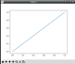x-axis

### Workflow

The basic steps to creating plots with matplotlib are:

1 Prepare Data
2 Create Plot
3 Plot
4 Customized Plot
5 Save Plot
6 Show Plot

``````>>> import matplotlib.pyplot as plt
>>> x = [1,2,3,4]  #Step 1
>>> y = [10,20,25,30]
>>> fig = plt.figure() #Step 2
>>> ax = fig.add_subplot(111) #Step 3
>>> ax.plot(x, y, color= 'lightblue', linewidth=3)  #Step 3, 4
>>> ax.scatter([2,4,6],
[5,15,25],
color= 'darkgreen',
marker= '^' )
>>> ax.set_xlim(1, 6.5)
>>> plt.savefig('foo.png' ) #Step 5
>>> plt.show() #Step 6``````

## Close and Clear

``````>>> plt.cla()  #Clear an axis
>>> plt.clf(). #Clear the entire figure
>>> plt.close(). #Close a window``````

## Plotting Customize Plot

### Colors, Color Bars & Color Maps

``````>>> plt.plot(x, x, x, x**2, x, x** 3)
>>> ax.plot(x, y, alpha = 0.4)
>>> ax.plot(x, y, c= 'k')
>>> fig.colorbar(im, orientation= 'horizontal')
>>> im = ax.imshow(img,
cmap= 'seismic' )``````

### Markers

``````>>> fig, ax = plt.subplots()
>>> ax.scatter(x,y,marker= ".")
>>> ax.plot(x,y,marker= "o")``````

### Linestyles

``````>>> plt.plot(x,y,linewidth=4.0)
>>> plt.plot(x,y,ls= 'solid')
>>> plt.plot(x,y,ls= '--')
>>> plt.plot(x,y,'--' ,x**2,y**2,'-.' )
>>> plt.setp(lines,color= 'r',linewidth=4.0)``````

### Text & Annotations

``````>>> ax.text(1,
-2.1,
'Example Graph',
style= 'italic' )
>>> ax.annotate("Sine",
xy=(8, 0),
xycoords= 'data',
xytext=(10.5, 0),
textcoords= 'data',
arrowprops=dict(arrowstyle= "->",
connectionstyle="arc3"),)``````

### Mathtext

``>>> plt.title(r '\$sigma_i=15\$', fontsize=20)``

### Limits, Legends and Layouts

Limits & Autoscaling

``````>>> ax.margins(x=0.0,y=0.1) #Add padding to a plot
>>> ax.axis('equal')  #Set the aspect ratio of the plot to 1
>>> ax.set(xlim=[0,10.5],ylim=[-1.5,1.5])  #Set limits for x-and y-axis
>>> ax.set_xlim(0,10.5) #Set limits for x-axis``````

Legends

``````>>> ax.set(title= 'An Example Axes',  #Set a title and x-and y-axis labels
ylabel= 'Y-Axis',
xlabel= 'X-Axis')
>>> ax.legend(loc= 'best')  #No overlapping plot elements``````

Ticks

``````>>> ax.xaxis.set(ticks=range(1,5),  #Manually set x-ticks
ticklabels=[3,100, 12,"foo" ])
>>> ax.tick_params(axis= 'y', #Make y-ticks longer and go in and out
direction= 'inout',
length=10)``````

Subplot Spacing

``````>>> fig3.subplots_adjust(wspace=0.5,   #Adjust the spacing between subplots
hspace=0.3,
left=0.125,
right=0.9,
top=0.9,
bottom=0.1)
>>> fig.tight_layout() #Fit subplot(s) in to the figure area``````

Axis Spines

``````>>> ax1.spines[ 'top'].set_visible(False) #Make the top axis line for a plot invisible
>>> ax1.spines['bottom' ].set_position(( 'outward',10))  #Move the bottom axis line outward``````

Have this Cheat Sheet at your fingertips

Original article source at https://www.datacamp.com

#matplotlib #cheatsheet #python1617634595

## Build Glass Website with HTML, CSS and Bootstrap Tutorial 2021

In this tutorial we will create a modern beautiful, video background glassmorphism landing page for a Travel website using HTML, CSS, Bootstrap 4 and a little JavaScript.

https://youtu.be/MsSlahoeeLo

#glassmorphism #glass morphism 2021 #how to make a website using html and css #how to create a website using html and css step by step website tutorial #video background website #glass website1603332000

## How WordPress Helps you Make Great Websites in 2020

Many sites are powered by a CMS (Content Management System) called WordPress. WordPress is an Open Source Software that offers free use of the product.

Before you launch, your WordPress site go through this checklist to get a better understanding

I will assume you have already purchased a domain name and had a hosting account set up with a service provider.

Many service providers are willing to go as far as installing WordPress for you now.

WordPress is great, but you must also learn about how websites work. Beginners should start by learning HTML (HyperText Markup Language) and CSS (Cascading Style Sheets).

These two computer languages basically cover how things are arranged and displayed on a page.

You should also understand how the FTP protocol is used for transferring files to your HTTP server.

Once you have got a good feel of HTML, CSS, HTTP, and FTP you will be ready to start exploring WordPress.

It allows you to change the look and feel of your website through a backside control panel.

You will find that WP is very robust and scalable.

It can provide a solution ranging from a single web page to an expansive multi-page website.

Another cool thing about WP is how it offers database solutions like pages, categories, comments, etc.

You can use it as a Blog or you can create static web pages. I am a huge fan. I have tried JOOMLA, but I still prefer WP.

There is a lot to learn when you are considering building a website.

I have been using WP for about 5 years and I am still learning. There is plenty of documentation available on the net.

If you are in need of a website, and you are starting this from scratch, I would recommend hiring a developer. But if you can accomplish learning this you will be able to build a good website solution for almost anyone.

#wordpress #wordpress-website #web-development #wordpress-website-building #wordpress-website-development #wordpress-tutorial #website #website-development1653464648

## Python Data Visualization: Bokeh Cheat Sheet

A handy cheat sheet for interactive plotting and statistical charts with Bokeh.

Bokeh distinguishes itself from other Python visualization libraries such as Matplotlib or Seaborn in the fact that it is an interactive visualization library that is ideal for anyone who would like to quickly and easily create interactive plots, dashboards, and data applications.

Bokeh is also known for enabling high-performance visual presentation of large data sets in modern web browsers.

For data scientists, Bokeh is the ideal tool to build statistical charts quickly and easily; But there are also other advantages, such as the various output options and the fact that you can embed your visualizations in applications. And let's not forget that the wide variety of visualization customization options makes this Python library an indispensable tool for your data science toolbox.

Now, DataCamp has created a Bokeh cheat sheet for those who have already taken the course and that still want a handy one-page reference or for those who need an extra push to get started.

In short, you'll see that this cheat sheet not only presents you with the five steps that you can go through to make beautiful plots but will also introduce you to the basics of statistical charts.

In no time, this Bokeh cheat sheet will make you familiar with how you can prepare your data, create a new plot, add renderers for your data with custom visualizations, output your plot and save or show it. And the creation of basic statistical charts will hold no secrets for you any longer.

Boost your Python data visualizations now with the help of Bokeh! :)

## Plotting With Bokeh

The Python interactive visualization library Bokeh enables high-performance visual presentation of large datasets in modern web browsers.

Bokeh's mid-level general-purpose bokeh. plotting interface is centered around two main components: data and glyphs.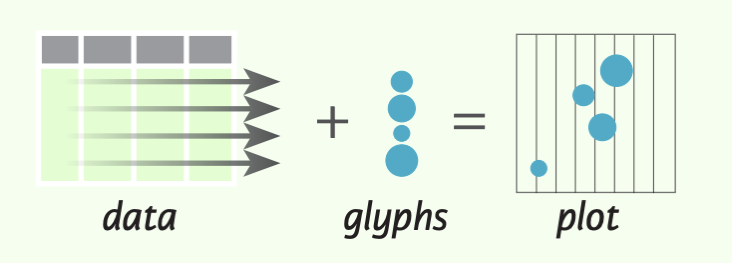The basic steps to creating plots with the bokeh. plotting interface are:

1. Prepare some data (Python lists, NumPy arrays, Pandas DataFrames and other sequences of values)
2. Create a new plot
4. Specify where to generate the output
5. Show or save the results
``````>>> from bokeh.plotting import figure
>>> from bokeh.io import output_file, show
>>> x = [1, 2, 3, 4, 5] #Step 1
>>> y = [6, 7, 2, 4, 5]
>>> p = figure(title="simple line example", #Step 2
x_axis_label='x',
y_axis_label='y')
>>> p.line(x, y, legend="Temp.", line_width=2) #Step 3
>>> output_file("lines.html") #Step 4
>>> show(p) #Step 5``````

## 1. Data

Under the hood, your data is converted to Column Data Sources. You can also do this manually:

``````>>> import numpy as np
>>> import pandas as pd
>>> df = pd.OataFrame(np.array([[33.9,4,65, 'US'], [32.4, 4, 66, 'Asia'], [21.4, 4, 109, 'Europe']]),
columns= ['mpg', 'cyl',   'hp',   'origin'],
index=['Toyota', 'Fiat', 'Volvo'])

>>> from bokeh.models import ColumnOataSource
>>> cds_df = ColumnOataSource(df)``````

## 2. Plotting

``````>>> from bokeh.plotting import figure
>>>p1= figure(plot_width=300, tools='pan,box_zoom')
>>> p2 = figure(plot_width=300, plot_height=300,
x_range=(0, 8), y_range=(0, 8))
>>> p3 = figure()``````

## 3. Renderers & Visual Customizations

### Glyphs

Scatter Markers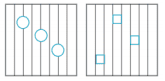``````>>> p1.circle(np.array([1,2,3]), np.array([3,2,1]), fill_color='white')
>>> p2.square(np.array([1.5,3.5,5.5]), [1,4,3],
color='blue', size=1)
``````

Line Glyphs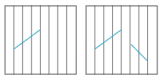``````>>> pl.line([1,2,3,4], [3,4,5,6], line_width=2)
>>> p2.multi_line(pd.DataFrame([[1,2,3],[5,6,7]]),
pd.DataFrame([[3,4,5],[3,2,1]]),
color="blue")
``````

### Customized Glyphs

Selection and Non-Selection Glyphs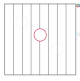``````>>> p = figure(tools='box_select')
>>> p. circle ('mpg', 'cyl', source=cds_df,
selection_color='red',
nonselection_alpha=0.1)
``````

Hover Glyphs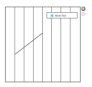``````>>> from bokeh.models import HoverTool
>>>hover= HoverTool(tooltips=None, mode='vline')
``````

Color Mapping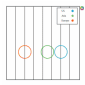``````>>> from bokeh.models import CategoricalColorMapper
>>> color_mapper = CategoricalColorMapper(
factors= ['US', 'Asia', 'Europe'],
palette= ['blue', 'red', 'green'])
>>>  p3. circle ('mpg', 'cyl', source=cds_df,
color=dict(field='origin',
transform=color_mapper), legend='Origin')
``````

## 4. Output & Export

### Notebook

``````>>> from bokeh.io import output_notebook, show
>>> output_notebook()
``````

### HTML

Standalone HTML

``````>>> from bokeh.embed import file_html
>>> from bokeh.resources import CON
>>> html = file_html(p, CON, "my_plot")

>>> from  bokeh.io  import  output_file,  show
>>> output_file('my_bar_chart.html',  mode='cdn')``````

Components

``````>>> from bokeh.embed import components
>>> script, div= components(p)``````

### PNG

``````>>> from bokeh.io import export_png
>>> export_png(p, filename="plot.png")``````

### SVG

``````>>> from bokeh.io import export_svgs
>>> p. output_backend = "svg"
>>> export_svgs(p,filename="plot.svg")``````

### Legend Location

Inside Plot Area

``````>>> p.legend.location = 'bottom left'
``````

Outside Plot Area

``````>>> from bokeh.models import Legend
>>> r1 = p2.asterisk(np.array([1,2,3]), np.array([3,2,1])
>>> r2 = p2.line([1,2,3,4], [3,4,5,6])
>>> legend = Legend(items=[("One" ,[p1, r1]),("Two",[r2])], location=(0, -30))

### Legend Background & Border

``````>>> p.legend. border_line_color = "navy"
>>> p.legend.background_fill_color = "white"``````

### Legend Orientation

``````>>> p.legend.orientation = "horizontal"
>>> p.legend.orientation = "vertical"``````

### Rows & Columns Layout

Rows

``````>>> from bokeh.layouts import row
>>>layout= row(p1,p2,p3)
``````

Columns

``````>>> from bokeh.layouts import columns
>>>layout= column(p1,p2,p3)``````

Nesting Rows & Columns

``````>>>layout= row(column(p1,p2), p3)
``````

### Grid Layout

``````>>> from bokeh.layouts import gridplot
>>> rowl = [p1,p2]
>>> row2 = [p3]
>>> layout = gridplot([[p1, p2],[p3]])``````

### Tabbed Layout

``````>>> from bokeh.models.widgets import Panel, Tabs
>>> tab1 = Panel(child=p1, title="tab1")
>>> tab2 = Panel(child=p2, title="tab2")
>>> layout = Tabs(tabs=[tab1, tab2])``````

``````Linked Axes
>>> p2.x_range = p1.x_range
>>> p2.y_range = p1.y_range``````

``````>>> p4 = figure(plot_width = 100, tools='box_select,lasso_select')
>>> p4.circle('mpg', 'cyl' , source=cds_df)
>>> p5 = figure(plot_width = 200, tools='box_select,lasso_select')
>>> p5.circle('mpg', 'hp', source=cds df)
>>>layout= row(p4,p5)``````

## 5. Show or Save Your Plots

``````>>> show(p1)
>>> show(layout)
>>> save(p1)``````

Have this Cheat Sheet at your fingertips

Original article source at https://www.datacamp.com

#python #datavisualization #bokeh #cheatsheet1618639156

## Responsive Personal Portfolio Website HTML CSS and JavaScript

#portfolio website html css #personal portfolio website tutorial #portfolio website #responsive personal portfolio website #portfolio website html css javascript #responsive portfolio website html css javascript